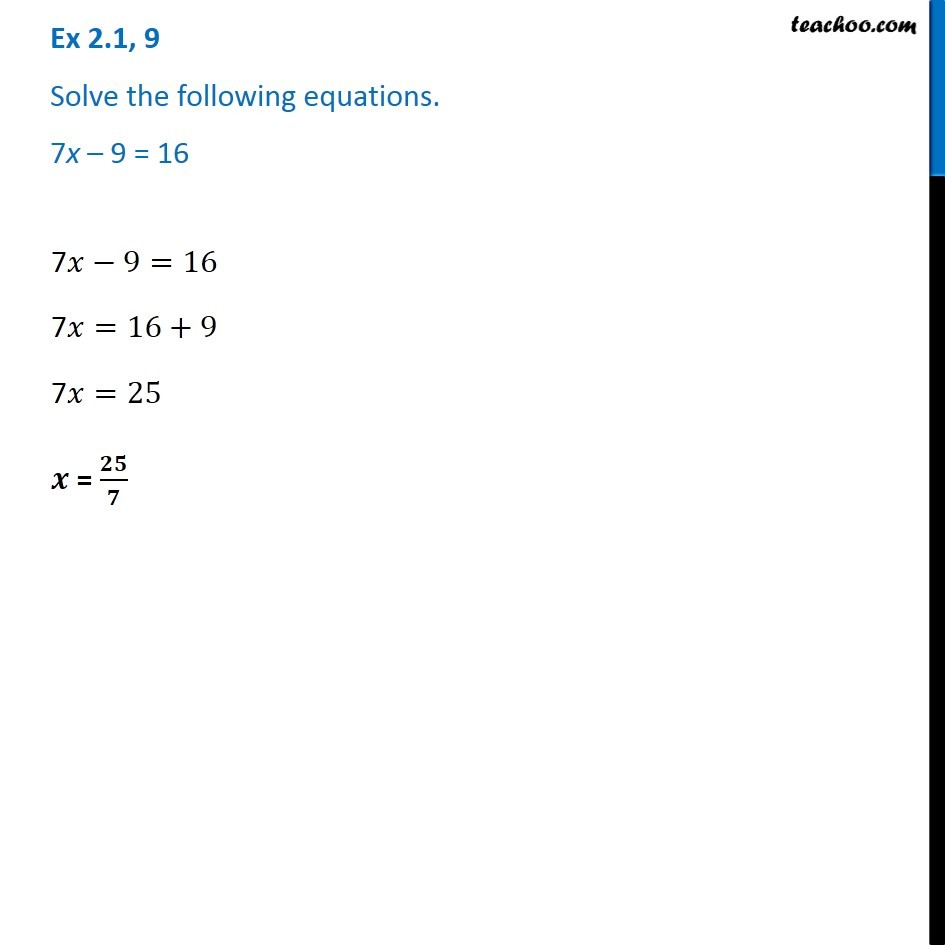Ex 2.1

Chapter 2 Class 8 Linear Equations in One Variable
Serial order wiseGet live Maths 1-on-1 Classs - Class 6 to 12

### Transcript

Ex 2.1, 9 - Chapter 2 Class 8 - Linear Equations in one variable - NCERT Book Solve the following equations. 7x – 9 = 16 7x − 9 = 16 7x = 16 + 9 7x = 25 x = 25/7